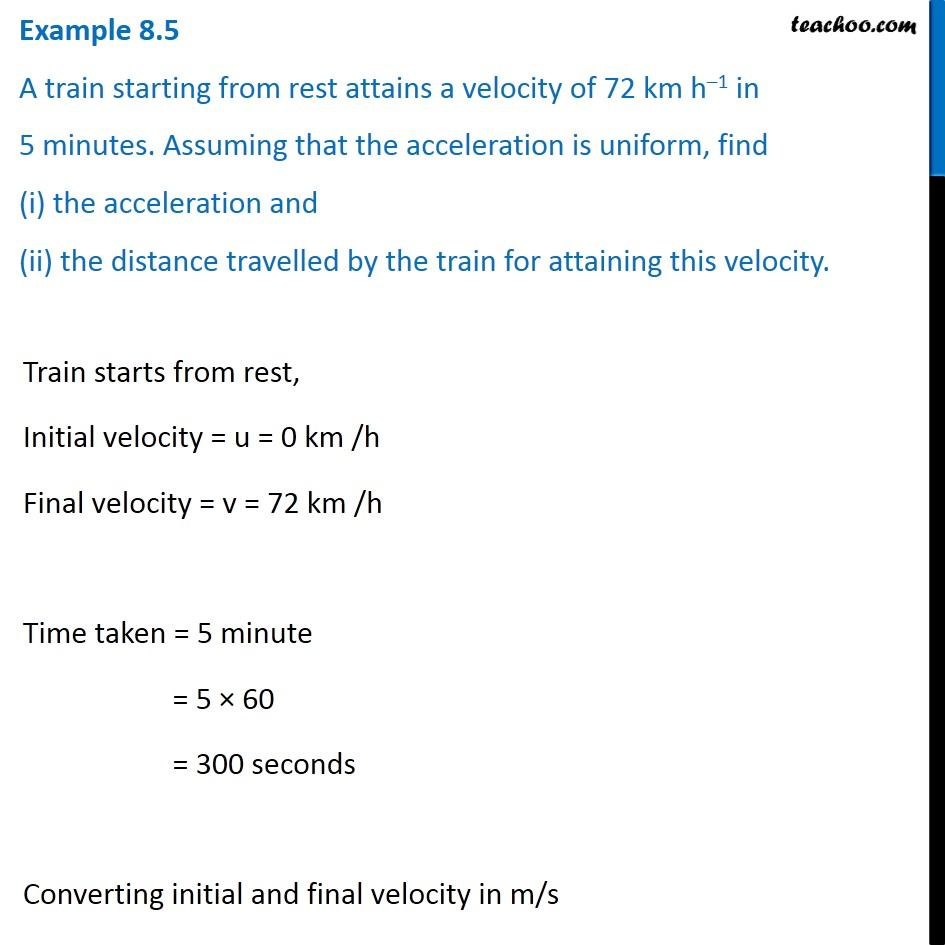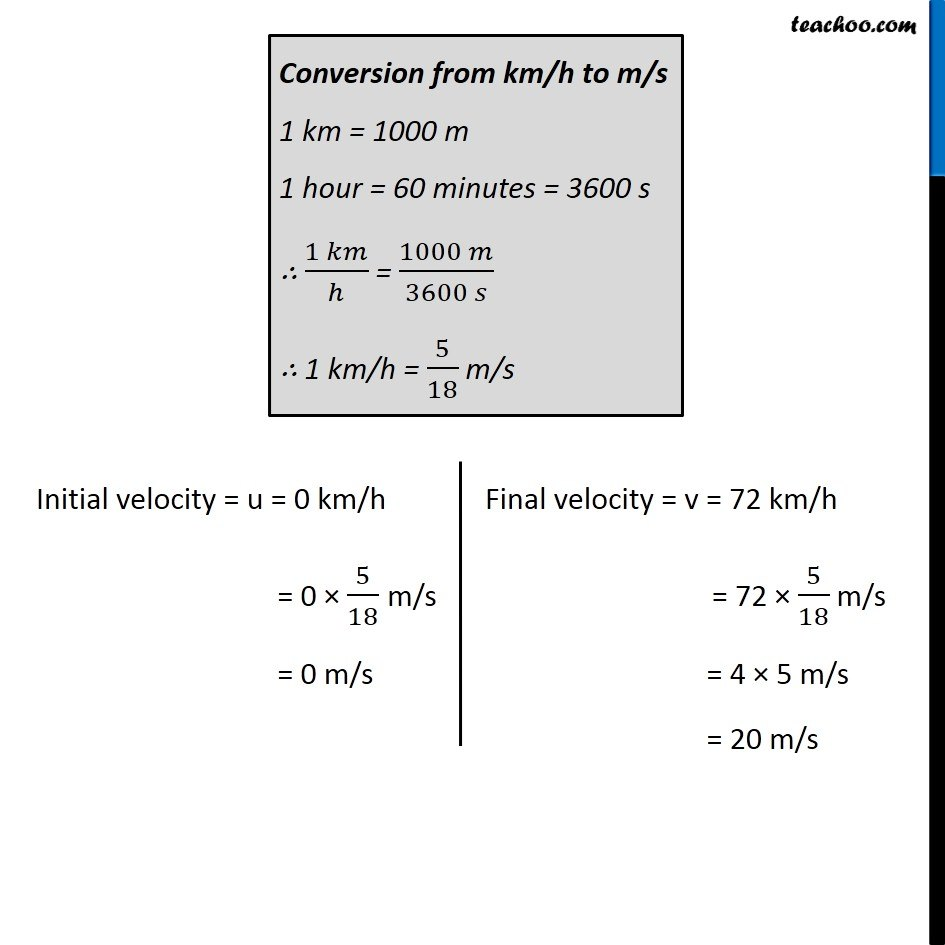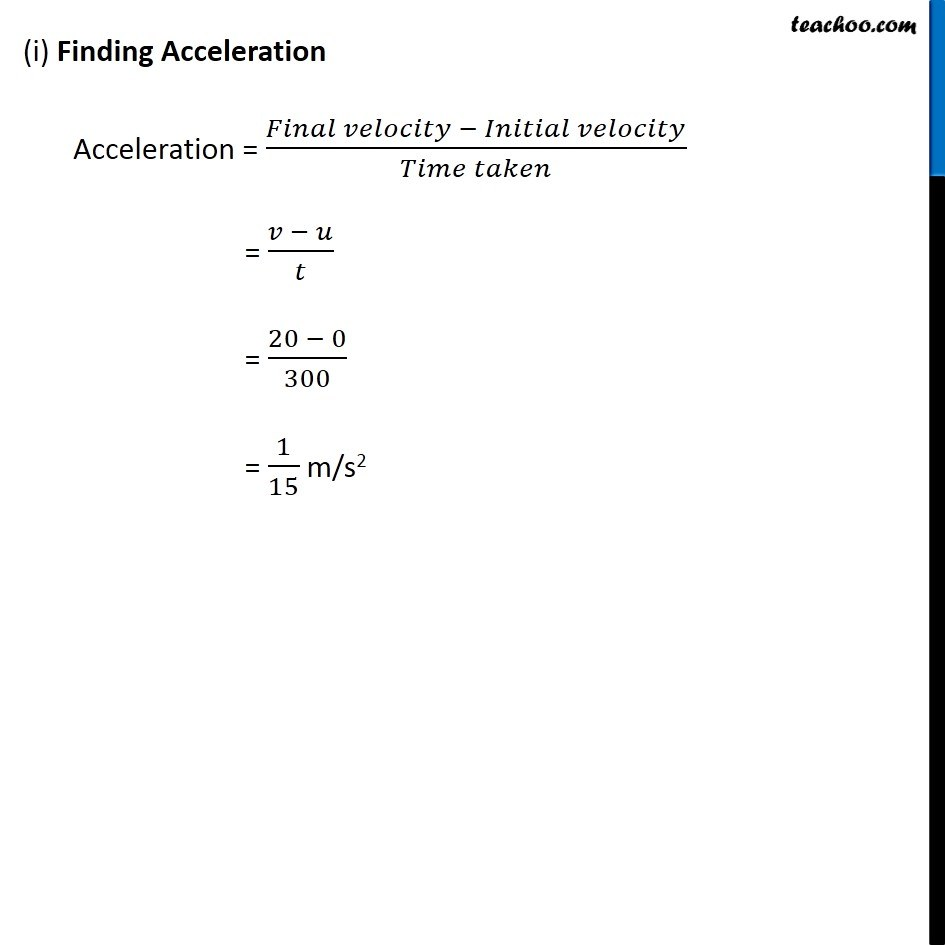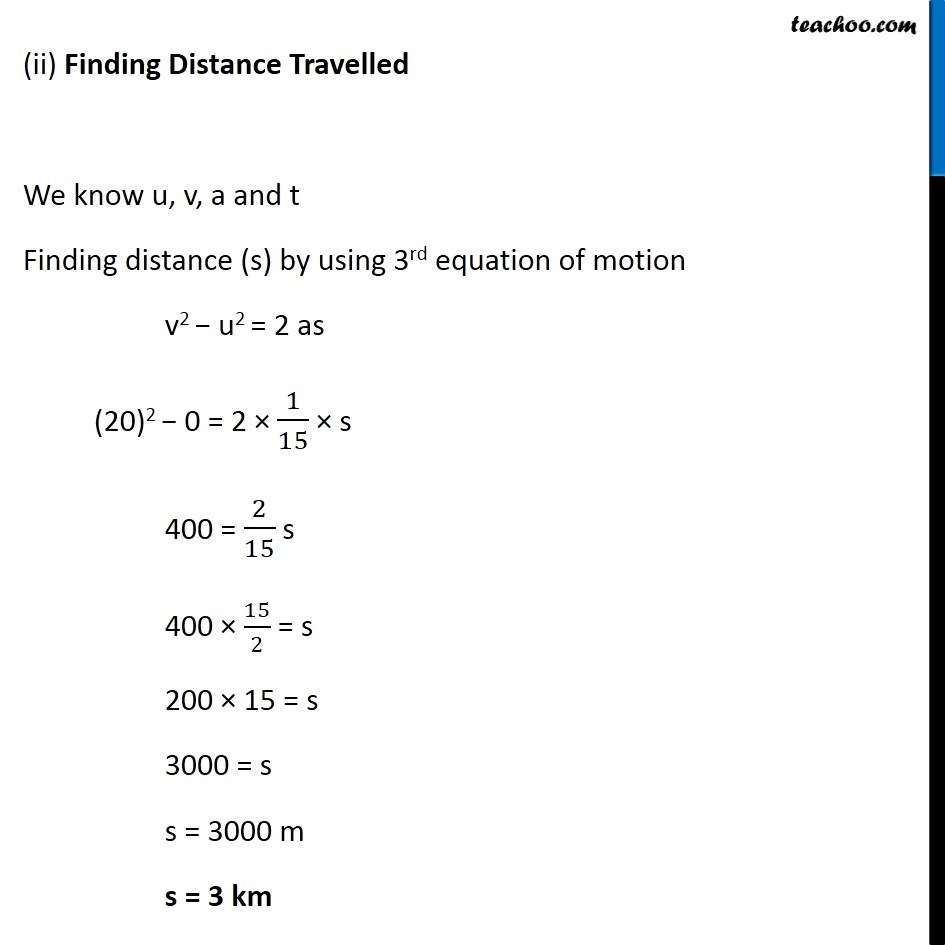Examples from NCERT Book

Class 9
Chapter 8 Class 9 - MotionLearn in your speed, with individual attention - Teachoo Maths 1-on-1 Class

### Transcript

Example 8.5 A train starting from rest attains a velocity of 72 km h–1 in 5 minutes. Assuming that the acceleration is uniform, find (i) the acceleration and (ii) the distance travelled by the train for attaining this velocity. Train starts from rest, Initial velocity = u = 0 km /h Final velocity = v = 72 km /h Time taken = 5 minute = 5 × 60 = 300 seconds Converting initial and final velocity in m/s Conversion from km/h to m/s 1 km = 1000 m 1 hour = 60 minutes = 3600 s ∴ (1 𝑘𝑚)/ℎ = (1000 𝑚)/(3600 𝑠) ∴ 1 km/h = 5/18 m/s Initial velocity = u = 0 km/h = 0 × 5/18 m/s = 0 m/s Final velocity = v = 72 km/h = 72 × 5/18 m/s = 4 × 5 m/s = 20 m/s (i) Finding Acceleration Acceleration = (𝐹𝑖𝑛𝑎𝑙 𝑣𝑒𝑙𝑜𝑐𝑖𝑡𝑦 − 𝐼𝑛𝑖𝑡𝑖𝑎𝑙 𝑣𝑒𝑙𝑜𝑐𝑖𝑡𝑦)/(𝑇𝑖𝑚𝑒 𝑡𝑎𝑘𝑒𝑛) = (𝑣 − 𝑢)/𝑡 = (20 − 0)/300 = 1/15 m/s2 (ii) Finding Distance Travelled We know u, v, a and t Finding distance (s) by using 3rd equation of motion v2 − u2 = 2 as (20)2 − 0 = 2 × 1/15 × s 400 = 2/15 s 400 × 15/2 = s 200 × 15 = s 3000 = s s = 3000 m s = 3 km